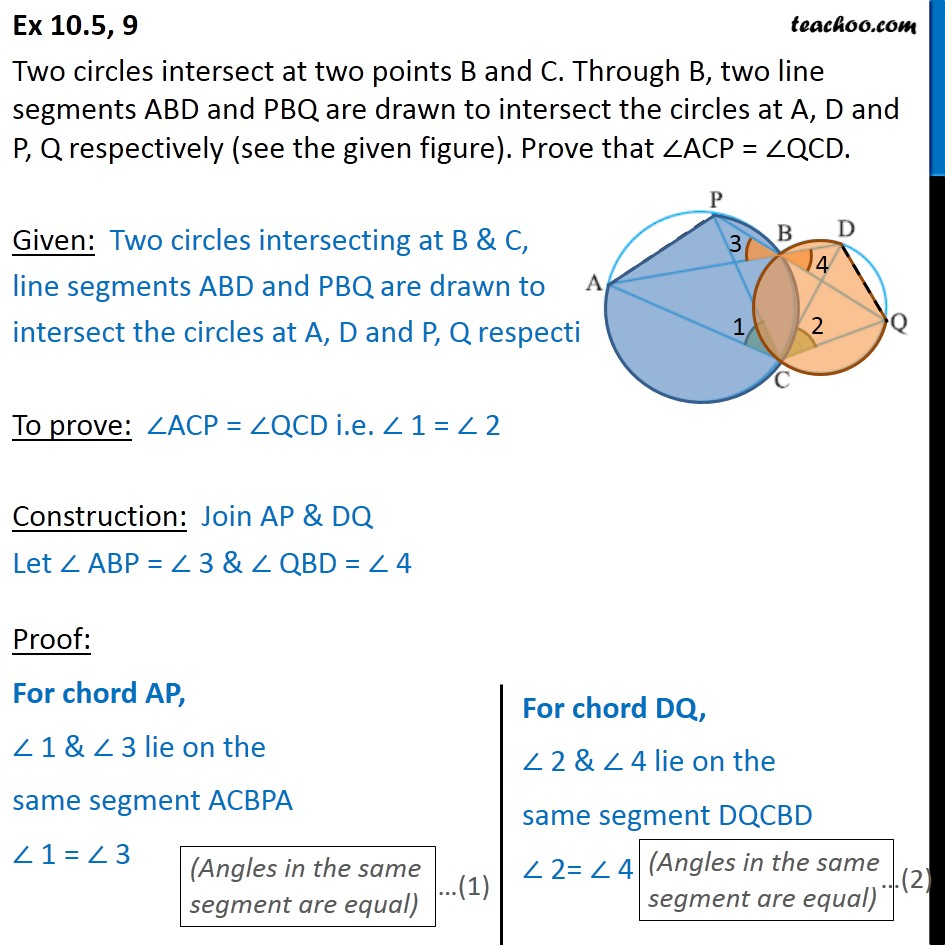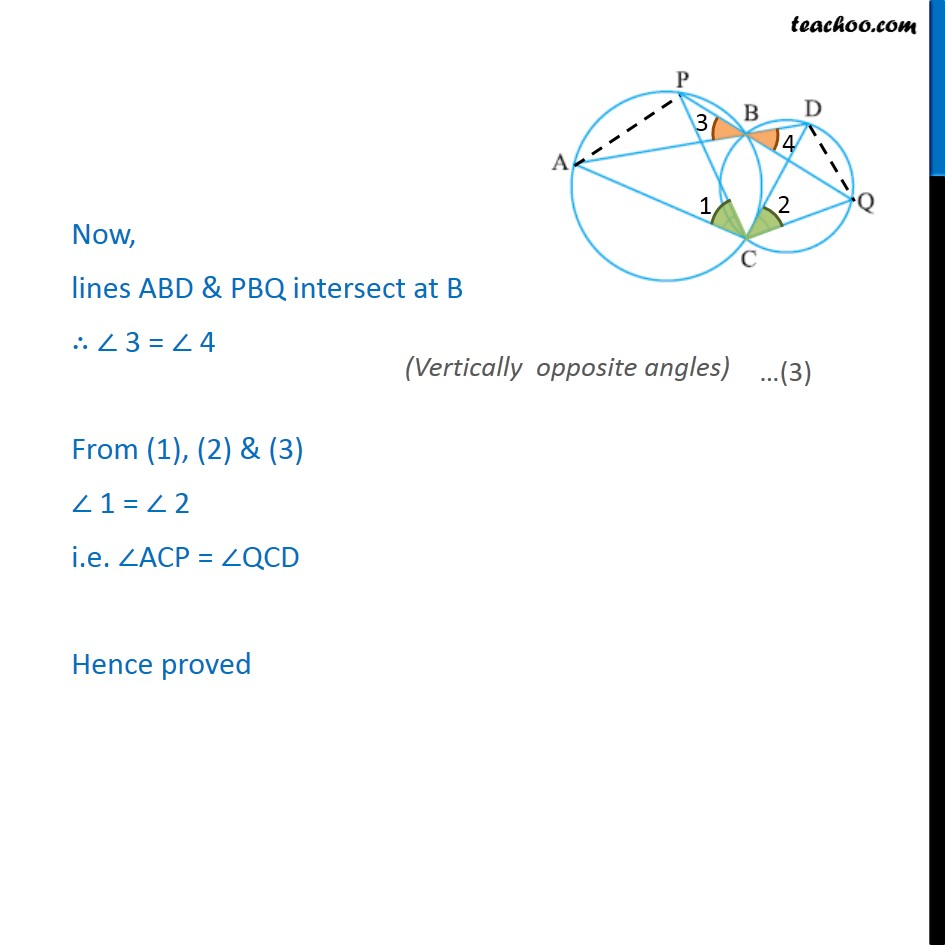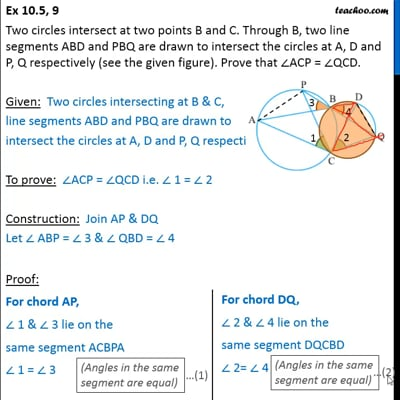Ex 10.5

Chapter 10 Class 9 Circles
Serial order wiseThis video is only available for Teachoo black users

Introducing your new favourite teacher - Teachoo Black, at only ₹83 per month

### Transcript

Ex 10.5, 9 Two circles intersect at two points B and C. Through B, two line segments ABD and PBQ are drawn to intersect the circles at A, D and P, Q respectively (see the given figure). Prove that ACP = QCD. Given: Two circles intersecting at B & C, line segments ABD and PBQ are drawn to intersect the circles at A, D and P, Q respectively To prove: ACP = QCD i.e. 1 = 2 Construction: Join AP & DQ Let ABP = 3 & QBD = 4 Proof: For chord AP, 1 & 3 lie on the same segment ACBPA 1 = 3 Now, lines ABD & PBQ intersect at B 3 = 4 From (1), (2) & (3) 1 = 2 i.e. ACP = QCD Hence proved